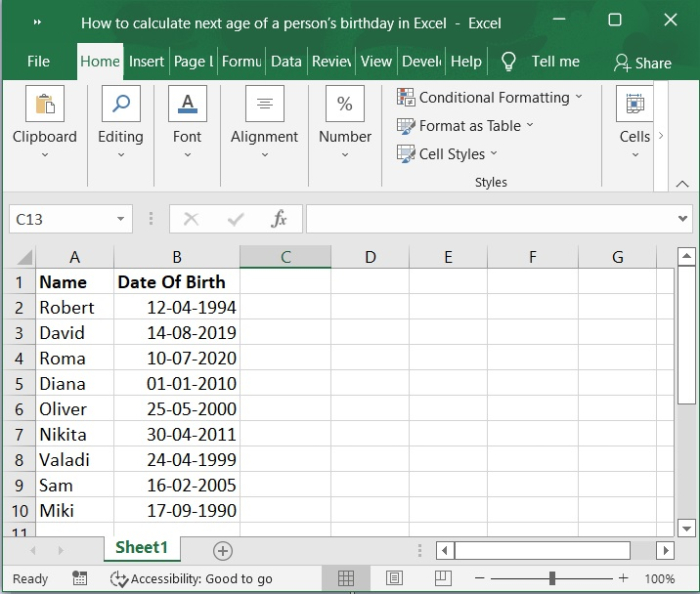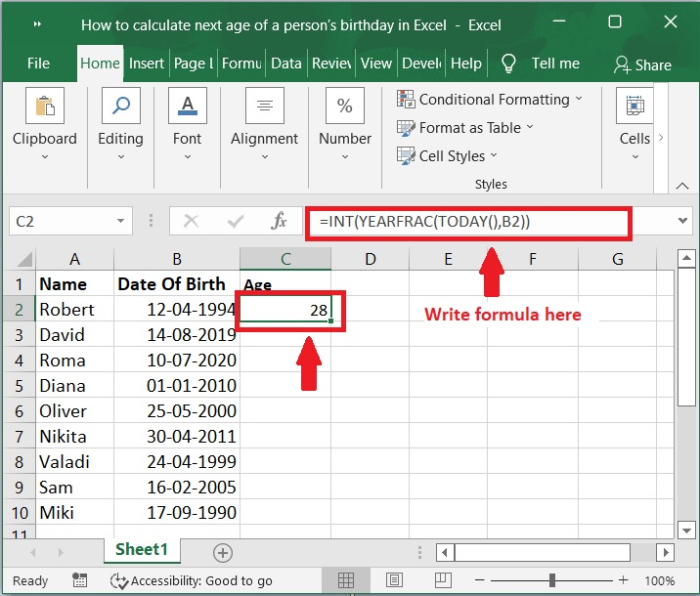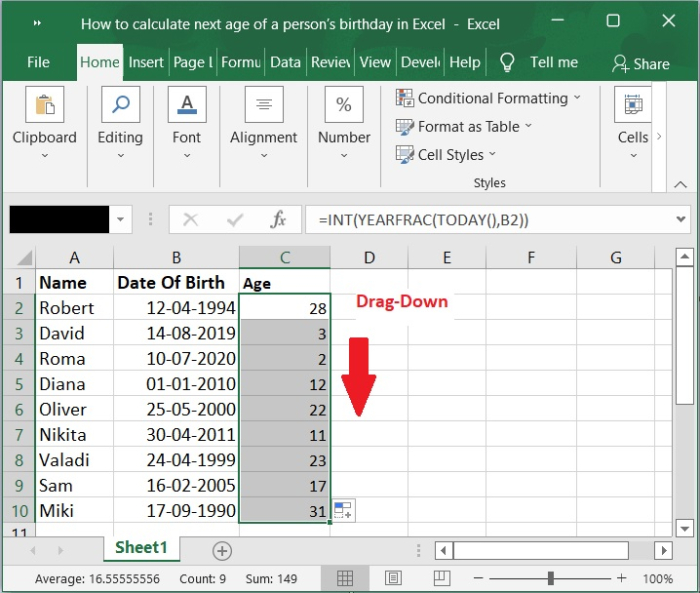# How to calculate the next age of a personâ€™s birthday in Excel?

When you ask someone in regular conversation, "How old are you?" the expectation is that they will provide a response that indicates the number of years they have been living. You are able to construct a formula in Microsoft Excel that can accurately compute an individual's age in months, days, hours, and even minutes. But since we're being traditional here, let's start with learning how to compute age based on date of birth in years.

If we know a person's birth date, we can use a formula to determine their age. However, have you ever considered using Excel to determine a person's age based on the age that they will be on their next birthday? That requires one to do the math to determine how old he will be on his next birthday. In this article, I will discuss different formulas that can be used to solve this problem using Excel.

In this tutorial, we will use a set of formula to determine how old a person will be on their next birthday. Letâ€™s understand step by step with an example.

## Step 1

In the first step, letâ€™s assume we have a sample data. Please refer to the below screenshot.## Step 2

If you wish to find out how old you will be on your next birthday, enter the formula that follows into a cell that is otherwise empty.

=INT(YEARFRAC(TODAY(),B2))## Step 3

After that, simply slide the fill handle down to the cells where you want the result to appear, and all of the ages in the following birthday depending on the birth date will be computed for you. Please refer to the below screenshot for the same.## Conclusion

In this tutorial, we used a simple example to demonstrate how you can use Excel to calculate how old a person will be on their next birthday.

Updated on: 03-Feb-2023

218 Views